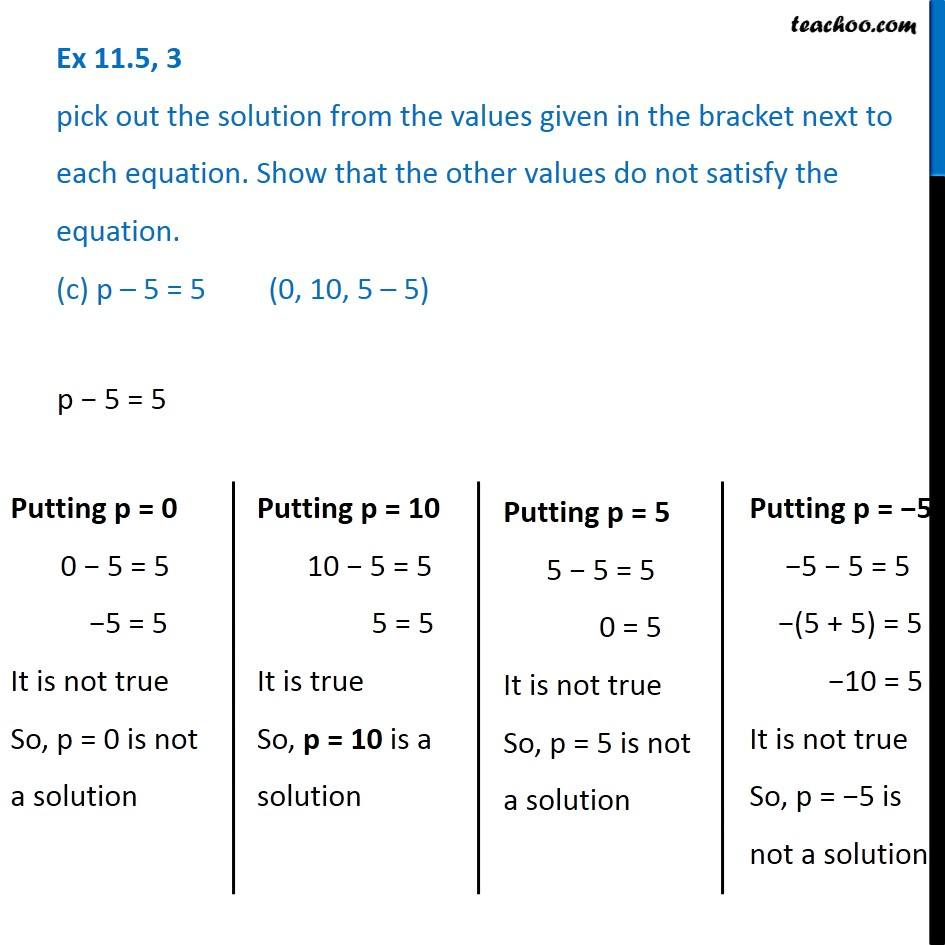Finding solutions of Algebra Equations - WorksheetLearn in your speed, with individual attention - Teachoo Maths 1-on-1 Class

### Transcript

Question 3 pick out the solution from the values given in the bracket next to each equation. Show that the other values do not satisfy the equation. (c) p – 5 = 5 (0, 10, 5 – 5)p − 5 = 5 Putting p = 0 0 − 5 = 5 −5 = 5 It is not true So, p = 0 is not a solution Putting p = 10 10 − 5 = 5 5 = 5 It is true So, p = 10 is a solution Putting p = 5 5 − 5 = 5 0 = 5 It is not true So, p = 5 is not a solution Putting p = −5 −5 − 5 = 5 −(5 + 5) = 5 −10 = 5 It is not true So, p = −5 is not a solution